Gaurav Singhal

# NMT: Encoder and Decoder with Keras

• Nov 19, 2020
• 9 Min read
• 7,472 Views
• Nov 19, 2020
• 9 Min read
• 7,472 Views

## Introduction

This guide builds on skills covered in Encoders and Decoders for Neural Machine Translation, which covers the different RNN models and the power of seq2seq modeling. It also covered the roles of encoder and decoder models in machine translation; they are two separate RNN models, combined to perform complex deep learning tasks.

By the end of the previous guide, you will have the pre-processed data and have extracted the features you need to build the model.

In this part of the guide, you will use that data and the concepts of LSTM, encoders, and decoders to build a network that gives optimum translation results. Finally, these results are further used to build a simple code to learn Spanish, which will give you random English sentences with their Spanish translations.

## Building the Model

The first step is to define an input sequence for the encoder. Because it's a character-level translation, it plugs the input into the encoder character by character. Now you need the encoder's final output as an initial state/input to the decoder. So, for the encoder LSTM model, the `return_state = True`. With this, you can get the hidden state representation of the encoder at the end of the input sequence. `state_h` denotes a hidden state and `state_c` denotes cell state.

``````1encoder_inputs = keras.Input(shape=(None, num_encoder_tokens))
2encoder = keras.layers.LSTM(latent_dim, return_state=True)
3encoder_outputs, state_h, state_c = encoder(encoder_inputs)
4
5encoder_states = [state_h, state_c]``````
python

This sets the initial state for the decoder in `decoder_inputs`. The first character got from one-hot encoding (`decoder_input_data`), i.e., SOS or `\t` is embedded with the final encoded state, to the decoder network to get the first target character.

Again, the LSTM `return_sequences` and `return_state` are kept `True` so that the network considers the decoder output and two decoder states at every time step. The model will run through each layer of the network, one step at a time, and add a `softmax` activation function at the last layer's output. This will give out your first output word. It feeds this word back and predicts the complete sentence.

``````1decoder_inputs = keras.Input(shape=(None, num_decoder_tokens))
2decoder_lstm = keras.layers.LSTM(latent_dim, return_sequences=True, return_state=True)
3decoder_outputs, _, _ = decoder_lstm(decoder_inputs, initial_state=encoder_states)
4decoder_dense = keras.layers.Dense(num_decoder_tokens, activation="softmax")
5decoder_outputs = decoder_dense(decoder_outputs)``````
python

## Training and Saving the Model

Now the aim is to train the basic LSTM-based seq2seq model and predict `decoder_target_data` and compile the model by setting the optimizer and learning rate, decay, and beta values. It calculates the loss and validation loss. Accuracy is the performance matrices. Next, fit the model, and split the data into an 80-20 ratio. And finally, use `save()` to save the model.

``````1model = keras.Model([encoder_inputs, decoder_inputs], decoder_outputs)
2
3model.compile(optimizer=Adam(lr=0.01, beta_1=0.9, beta_2=0.999, decay=0.001), loss='categorical_crossentropy', metrics=["accuracy"])
4
5model.fit(
6    [encoder_input_data, decoder_input_data],
7    decoder_target_data,
8    batch_size=batch_size,
9    epochs=epochs,
10    validation_split=0.2,
11)
12model.save("E2S")``````
python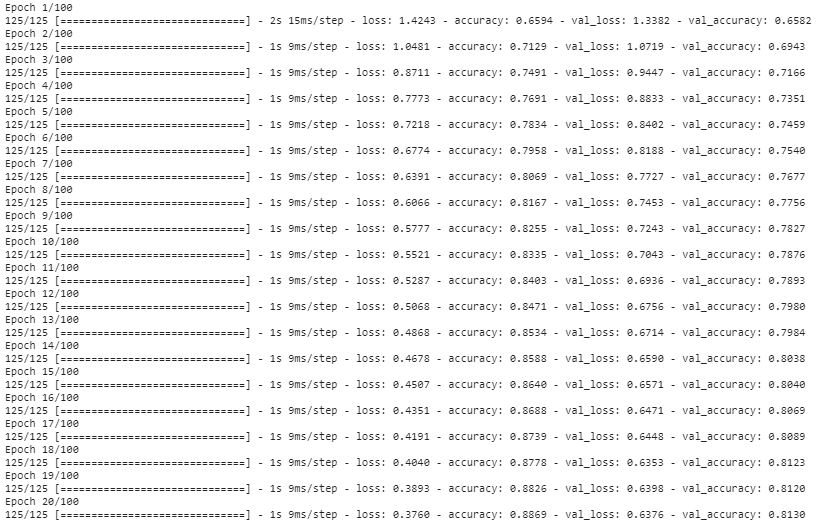``````1from keras.utils import plot_model
2plot_model(model, to_file='modelsummary.png', show_shapes=True, show_layer_names=True)``````
python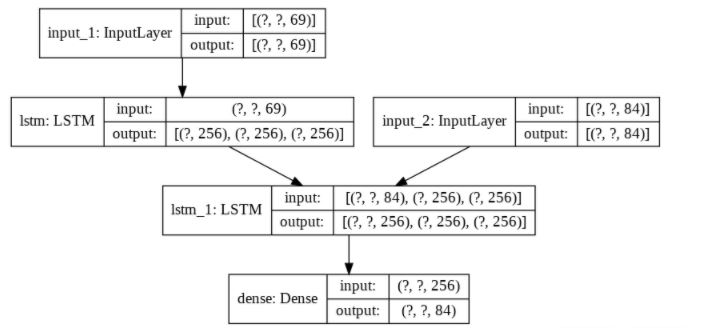``````1print("shape encoder_input_data :",encoder_input_data.shape)
2print("shape decoder_input_data :",decoder_input_data.shape)
3print("shape decoder_target_data:",decoder_target_data.shape)``````
python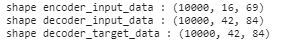## Decode the Sentence

Finally, create the model by using Keras `model()` function for `encoder_inputs` i.e., input tensor and encoder hidden states `state_h_enc` and `state_c_enc` as output tensor.

``````1encoder_inputs = model.input  # input_1
2encoder_outputs, state_h_enc, state_c_enc = model.layers.output  # lstm_1
3encoder_states = [state_h_enc, state_c_enc]
4encoder_model = keras.Model(encoder_inputs, encoder_states)``````
python

Now build the model for the decoder.

``````1decoder_inputs = model.input  # input_2
2decoder_state_input_h = keras.Input(shape=(latent_dim,), name="input_3")
3decoder_state_input_c = keras.Input(shape=(latent_dim,), name="input_4")
4decoder_states_inputs = [decoder_state_input_h, decoder_state_input_c]
5decoder_lstm = model.layers
6decoder_outputs, state_h_dec, state_c_dec = decoder_lstm(
7    decoder_inputs, initial_state=decoder_states_inputs
8)
9decoder_states = [state_h_dec, state_c_dec]
10decoder_dense = model.layers
11decoder_outputs = decoder_dense(decoder_outputs)
12decoder_model = keras.Model(
13    [decoder_inputs] + decoder_states_inputs, [decoder_outputs] + decoder_states
14)``````
python

Create two reverse-lookup token indexes to decode the sequence to make it readable.

``````1reverse_input_char_index = dict((i, char) for char, i in input_token_index.items())
2reverse_target_char_index = dict((i, char) for char, i in target_token_index.items())``````
python

Next, create a predict function named `decode_sequence`. After generating the empty sequence of length `1`, the model should know when to start and stop reading the text. To read the model will check out for `\t` in this case. Keep two conditions, either when the max length of sentence is hit or find stop character `\n`. Keep on updating the target sequence by one and update the states.

``````1def decode_sequence(input_seq):
2    states_value = encoder_model.predict(input_seq)
3
4    target_seq = np.zeros((1, 1, num_decoder_tokens))
5    target_seq[0, 0, target_token_index["\t"]] = 1.0
6
7    stop_condition = False
8    decoded_sentence = ""
9    while not stop_condition:
10        output_tokens, h, c = decoder_model.predict([target_seq] + states_value)
11
12        sampled_token_index = np.argmax(output_tokens[0, -1, :])
13        sampled_char = reverse_target_char_index[sampled_token_index]
14        decoded_sentence += sampled_char
15
16        if sampled_char == "\n" or len(decoded_sentence) > max_decoder_seq_length:
17            stop_condition = True
18
19        target_seq = np.zeros((1, 1, num_decoder_tokens))
20        target_seq[0, 0, sampled_token_index] = 1.0
21
22        states_value = [h, c]
23    return decoded_sentence``````
python

## Learn Spanish

A random sentence will appear when you run the cell. The sentences are basic. It's always an add-on to your skills to learn a new foreign language. Also, it will be helpful when you visit Spain :)

``````1i = np.random.choice(len(input_texts))
2input_seq = encoder_input_data[i:i+1]
3translation = decode_sequence(input_seq)
4print('-')
5print('Input:', input_texts[i])
6print('Translation:', translation)``````
python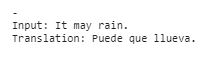Validate with google translator.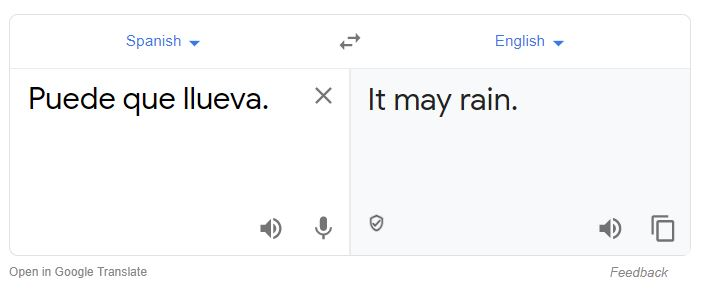Perfecto!!

## Conclusion

The character-by-character translation is accurate. Seq2seq models can deal with variable-length inputs. Encoders and decoders work together. Encoders' LSTM weights are updated so they learn space representation of the text, whereas decoders' LSTM weights give grammatically correct sentences. The performance of any project depends on the model you choose and the volume and pre-processing of the data. But hyper-parameters also play a major role in deep learning problems. You can improve the accuracy of this model as well by tuning the hyper-parameters or increasing the data.

Machine translation can also be performed by using the GRU RNN model. It's a cousin to LSTM with fewer states. I would recommend that you understand different RNN models. You can learn more about GRU here and learn to understand the difference between the two RNNs and select the model that gives you the best results.

If you have any queries regarding this guide, feel free to ask at Codealphabet.